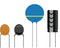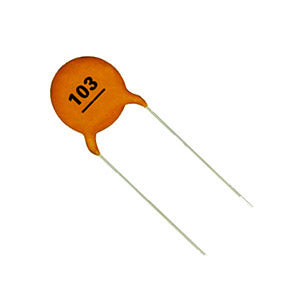Ceramic disc capacitors like the one on the left are printed with a code. This code indicates what the capacitance of the capacitor is. The example capacitor has a 3 digit number printed on it (103). The first two digits, in this case the 10 give us the first part of the value. The third digit indicates the number of extra zeros, in this case 3 extra zeros. So the value is 10 with 3 extra zeros, or 10,000.Ceramic disc capacitor codes are always measured in pico Farads or pF. So it is a 10,000pF capacitor.

When a capacitor can be written without needing any extra zeros to be added to the value, the third digit is not printed. If a capacitor only has two digits on it, say “10” then that is its value in pF. If a capacitor only has two digits on it, say “10” then that is its value in pF.   Download a pdf version of this page hereLearn more about the author